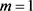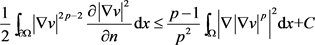﻿ 具有间接吸引信号产出的高维趋化增长系统解的有界性

# 具有间接吸引信号产出的高维趋化增长系统解的有界性Boundedness in the Higher-Dimensional Chemotaxis-Growth System with Indirect Attractant Production，其中是一个光滑有界区域，τ>0。利用能量方法和Moser迭代证明了在任意充分光滑的初值边界条件下，当µ足够大，该模型有唯一的全局有界经典解。

Abstract: This paper deals with the following Chemotaxis-growth system of the Mountain Pain Beetle with in-direct attractant production:in a smoothly bounded domain,   is positive. The energy method and Moser iteration are used to prove that under any sufficiently smooth initial boundary conditions, when µ is large enough, the model has a unique global-in-time classical solution.

Abstract:

1. 引言

$\left\{\begin{array}{cc}{u}_{t}=\Delta u-\nabla \cdot \left(u\nabla v\right)\text{+}\mu \left(u-{u}^{2}\right),& x\in \Omega ,t>0,\\ {v}_{t}=\Delta v+w-v,& x\in \Omega ,t>0,\\ \tau {w}_{t}+w=u,& x\in \Omega ,t>0,\\ \frac{\partial u}{\partial n}=\frac{\partial v}{\partial n}=\frac{\partial w}{\partial n}=0,& x\in \partial \Omega ,t>0,\\ u\left(x,0\right)={u}_{0}\left(x\right),\text{\hspace{0.17em}}v\left(x,0\right)={v}_{0}\left(x\right)\text{\hspace{0.17em}},w\left(x,0\right)={w}_{0}\left(x\right),& x\in \Omega ,\end{array}$ (1.1)

$\left\{\begin{array}{cc}{u}_{t}=\Delta u-\nabla \cdot \left(u\nabla v\right),& x\in \Omega ,t>0,\\ {v}_{t}=\Delta v-v+u,& x\in \Omega ,t>0\\ \frac{\partial u}{\partial n}=\frac{\partial v}{\partial n}=0,& x\in \partial \Omega ,t>0,\\ u\left(x,0\right)={u}_{0}\left(x\right),\text{\hspace{0.17em}}v\left(x,0\right)={v}_{0}\left(x\right),& x\in \Omega ,\end{array}$

$n=3$$\mu >0$ 时，Hu和Tao在文献  中证明了模型解的全局有界性。本文证明了 $n\ge 3$$\mu >{\delta }_{2}+C\left(p\right)\left(2{\delta }_{1}+\epsilon \right)$ 时，模型(1.1)解的全局有界性，其中 ${\delta }_{1}$${\delta }_{2}$$C\left(p\right)$ 满足(3.7)，(3.11)，(3.9)。当 $n\ge 2$ 时，Li和Tao  研究了logistic源为 $u-{u}^{\alpha }$ 时的情况，系数满足 $\alpha >n/2$ 时模型解的全局有界性，维数 $n\ge 4$ 时，显然 $\alpha >2$ ，而本文 $\alpha =2$ 优于其结果。在Hu和Tao研究的基础上，Qiu，Mu和Wang  将飞行甲壳虫的随机扩散项 $\Delta u$ 用非线性函数 $D\left(u\right)$ 来描述，考虑扩散系数 $D\left(u\right)$ 对模型的影响，当 $n\ge 3$ 时，假设扩散系数 $D\left(u\right)>{D}_{0}{u}^{\theta }$ 得到 $\theta >1-4/n$ 时模型解的全局有界性。而当 $n\ge 4$ 时，本文 $\theta =0$ 优于其结果。当 $n\ge 1$ 时，Zheng在文献  中假设扩散系数 $D\left(u\right)\ge {C}_{D}{\left(u+1\right)}^{m-1}$ 得到 $m>\left\{\begin{array}{cc}1-\frac{\mu }{\chi \left[1+{\lambda }_{0}{‖{v}_{0}‖}_{{L}^{\infty }\left(\Omega \right)}{2}^{3}\right]}& n\le 2,\\ 1& n\ge 3,\end{array}$ 时模型解的全局有界性。但是本文 $n\ge 3$是文献  的一个临界情况。

$\begin{array}{l}u\in {C}^{0}\left(\stackrel{¯}{\Omega }×\left[0,T{}_{\mathrm{max}}\right)\right)\cap {C}^{2,1}\left(\stackrel{¯}{\Omega }×\left(0,{T}_{\mathrm{max}}\right)\right),\\ v\in {C}^{0}\left(\stackrel{¯}{\Omega }×\left[0,T{}_{\mathrm{max}}\right)\right)\cap {C}^{2,1}\left(\stackrel{¯}{\Omega }×\left(0,{T}_{\mathrm{max}}\right)\right),\\ w\in {C}^{0,1}\left(\stackrel{¯}{\Omega }×\left[0,T{}_{\mathrm{max}}\right)\right),\end{array}$

$\Omega ×\left(0,\infty \right)$ 是模型(1.1)的经典解。特别的，存在一个常数 $C>0$ ，使得

$u\left(x,t\right)\le C$$v\left(x,t\right)\le C$$w\left(x,t\right)\le C,x\in \Omega$$t>0$

2. 先验估计

${‖\nabla v‖}_{{L}^{2p+2}\left(\Omega \right)}^{2p+2}\le {k}_{0}\left({‖{|\nabla v|}^{p-1}{D}^{2}v‖}_{{L}^{2}\left(\Omega \right)}^{2}+1\right)$

${\int }_{\Omega }u\left(\cdot ,t\right)\text{d}x\le C$${‖v\left(\cdot ,t\right)‖}_{{W}^{1,q}\left(\Omega \right)}\le C$${\int }_{\Omega }{u}^{2}\left(\cdot ,t\right)\text{d}x\le C$

3. 主要结论的证明

${‖u‖}_{{L}^{p}\left(\Omega \right)}^{p}\le C$${‖\nabla v‖}_{{L}^{2p}\left(\Omega \right)}^{2p}\le C$${‖w‖}_{{L}^{p\text{+}1}\left(\Omega \right)}^{p\text{+}1}\le C$

 (3.1)

$\frac{1}{2}{\int }_{\partial \Omega }{|\nabla v|}^{2p-2}\frac{\partial {|\nabla v|}^{2}}{\partial n}\text{\hspace{0.17em}}\text{d}x\le \frac{1}{2}{C}_{\Omega }{‖{|\nabla v|}^{p}‖}_{{L}^{2}\left(\partial \Omega \right)}^{2}\le {C}_{1}{‖{|\nabla v|}^{p}‖}_{{W}^{r+\frac{1}{2},2}\left(\Omega \right)}^{2}$(3.2)

${‖{|\nabla v|}^{p}‖}_{{W}^{r+\frac{1}{2},2}\left(\Omega \right)}^{2}\le {C}_{2}{‖{|\nabla v|}^{p}‖}_{{L}^{2}\left(\Omega \right)}^{a}\text{+}{C}_{3}\le \frac{p-1}{{C}_{1}{p}^{2}}{\int }_{\Omega }{|\nabla {|\nabla v|}^{p}|}^{2}\text{d}x\text{+}{C}_{4}$。 (3.3)

$\begin{array}{c}-{\int }_{\Omega }w\nabla v\cdot \nabla \left({|\nabla v|}^{2p-2}\right)\text{\hspace{0.17em}}\text{d}x=-\left(p-1\right){\int }_{\Omega }w{|\nabla v|}^{2\left(p-2\right)}\nabla v\cdot \nabla {|\nabla v|}^{2}\text{d}x\\ \le \frac{p-1}{4}{\int }_{\Omega }{|\nabla v|}^{2p-4}{|\nabla {|\nabla v|}^{2}|}^{2}dx+\left(p-1\right){\int }_{\Omega }{w}^{2}{|\nabla v|}^{2p-2}\text{d}x\\ \le \frac{p-1}{{p}^{2}}{\int }_{\Omega }{|\nabla {|\nabla v|}^{p}|}^{2}\text{d}x\text{+}\left(p-1\right){\int }_{\Omega }{w}^{2}{|\nabla v|}^{2p-2}\text{d}x.\end{array}$ (3.4)

$-{\int }_{\Omega }w{|\nabla v|}^{2p-2}\Delta v\text{d}x\le \sqrt{n}{\int }_{\Omega }w{|\nabla v|}^{2p-2}|{D}^{2}v|\text{ }\text{d}x\le \frac{1}{4}{\int }_{\Omega }{|\nabla v|}^{2p-2}{|{D}^{2}v|}^{2}\text{d}x\text{+}n{\int }_{\Omega }{w}^{2}{|\nabla v|}^{2p-2}\text{d}x$(3.5)

$\begin{array}{c}{\int }_{\Omega }{w}^{2}{|\nabla v|}^{2p-2}\text{d}x\le \frac{1}{4\left(n+p-1\right){k}_{0}}{\int }_{\Omega }{|\nabla v|}^{2p+2}\text{d}x+\frac{2}{p+1}{\left(\frac{4\left(n+p-1\right)\left(p-1\right){k}_{0}}{p+1}\right)}^{\frac{p-1}{2}}{\int }_{\Omega }{w}^{p+1}\text{d}x\\ \text{ }\le \frac{1}{4\left(n+p-1\right)}{\int }_{\Omega }{|\nabla v|}^{2p-2}{|{D}^{2}v|}^{2}\text{d}x+{\delta }_{1}{\int }_{\Omega }{w}^{p+1}\text{d}x+C，\end{array}$ (3.6)

$\frac{1}{2p}\frac{\text{d}}{\text{d}t}{‖\nabla v‖}_{{L}^{2p}\left(\Omega \right)}^{2p}+{\int }_{\Omega }{|\nabla v|}^{2p}\text{d}x+\frac{1}{2}{\int }_{\Omega }{|\nabla v|}^{2p-2}{|{D}^{2}v|}^{2}\text{d}x\le {\delta }_{1}{\int }_{\Omega }{w}^{p+1}\text{d}x+C,$ (3.7)

$\frac{\tau }{p+1}\frac{\text{d}}{\text{d}t}{‖w‖}_{{L}^{p+1}\left(\Omega \right)}^{p+1}+{\int }_{\Omega }{w}^{p+1}\text{d}x={\int }_{\Omega }u{w}^{p}\text{d}x\le \frac{1}{2}{\int }_{\Omega }{w}^{p+1}\text{d}x+C\left(p\right){\int }_{\Omega }{u}^{p+1}\text{d}x$(3.8)

$\frac{\tau }{p+1}\frac{\text{d}}{\text{d}t}{‖w‖}_{{L}^{p+1}\left(\Omega \right)}^{p+1}+\frac{1}{2}{\int }_{\Omega }{w}^{p+1}\text{d}x\le C\left(p\right){\int }_{\Omega }{u}^{p+1}\text{d}x$(3.9)

$\frac{1}{p}\frac{\text{d}}{\text{d}t}{‖u‖}_{{L}^{p}\left(\Omega \right)}^{p}+\left(p-1\right){\int }_{\Omega }{u}^{p-2}{|\nabla u|}^{2}\text{d}x=-{\int }_{\Omega }\nabla \cdot \left(u\nabla v\right){u}^{p-1}\text{d}x+\mu {\int }_{\Omega }{u}^{p}\text{d}x-\mu {\int }_{\Omega }{u}^{p+1}\text{d}x.$ (3.10)$\frac{1}{p}\frac{\text{d}}{\text{d}t}{‖u‖}_{{L}^{p}\left(\Omega \right)}^{p}\text{+}\frac{1}{p}{‖u‖}_{{L}^{p}\left(\Omega \right)}^{p}\le \frac{1}{2}{\int }_{\Omega }{|\nabla v|}^{2p-2}{|{D}^{2}v|}^{2}\text{d}x\text{+}\left(\mu +\frac{1}{p}\right){\int }_{\Omega }{u}^{p}\text{d}x-\left(\mu -{\delta }_{2}\right){\int }_{\Omega }{u}^{p+1}\text{d}x+C.$ (3.11)

$\begin{array}{l}\frac{\text{d}}{\text{d}t}\left[\frac{1}{2p}{‖\nabla v‖}_{{L}^{2p}\left(\Omega \right)}^{2p}+\frac{\tau \left(2{\delta }_{1}+\epsilon \right)}{p+1}{‖w‖}_{{L}^{p+1}\left(\Omega \right)}^{p+1}+\frac{1}{p}{‖u‖}_{{L}^{p}\left(\Omega \right)}^{p}\right]+{\int }_{\Omega }{|\nabla v|}^{2p}\text{d}x+\frac{\epsilon }{2}{\int }_{\Omega }{w}^{p+1}\text{d}x+\frac{1}{p}{‖u‖}_{{L}^{p}\left(\Omega \right)}^{p}\\ \le \left(\mu +\frac{1}{p}\right){\int }_{\Omega }{u}^{p}\text{d}x-\left[\mu -{\delta }_{2}-C\left(p\right)\left(2{\delta }_{1}+\epsilon \right)\right]{\int }_{\Omega }{u}^{p+1}\text{d}x+C,\end{array}$

${‖\nabla v‖}_{{L}^{2p}\left(\Omega \right)}^{2p}\le C$${‖w‖}_{{L}^{p+1}\left(\Omega \right)}^{p+1}\le C$${‖u‖}_{{L}^{p}\left(\Omega \right)}^{p}\le C$

${‖\nabla v\left(\cdot ,t\right)‖}_{{L}^{\infty }\left(\Omega \right)}\le C$

${‖u\left(\cdot ,t\right)‖}_{{L}^{\infty }\left(\Omega \right)}\le C$${‖w\left(\cdot ,t\right)‖}_{{L}^{\infty }\left(\Omega \right)}\le C$

$\begin{array}{c}\frac{1}{p}\frac{\text{d}}{\text{d}t}{‖u‖}_{{L}^{p}\left(\Omega \right)}^{p}+\left(p-1\right){\int }_{\Omega }{u}^{p-2}{|\nabla u|}^{2}\text{d}x\le \left(p-1\right){\int }_{\Omega }{u}^{p-1}|\nabla u||\nabla v|\text{d}x+\mu {\int }_{\Omega }{u}^{p-1}\left(u-{u}^{2}\right)\text{\hspace{0.17em}}\text{d}x\\ \le C{\int }_{\Omega }{u}^{p-1}|\nabla u|\text{\hspace{0.17em}}\text{d}x+\mu {\int }_{\Omega }{u}^{p-1}\left(u-{u}^{2}\right)\text{\hspace{0.17em}}\text{d}x\le \frac{p-1}{4}{\int }_{\Omega }{u}^{p-2}{|\nabla u|}^{2}\text{d}x\\ +C{\int }_{\Omega }{u}^{p}\text{d}x+\mu {\int }_{\Omega }{u}^{p-1}\left(u-{u}^{2}\right)\text{\hspace{0.17em}}\text{d}x\\ \le \frac{p-1}{4}{\int }_{\Omega }{u}^{p-2}{|\nabla u|}^{2}\text{d}x+C{\int }_{\Omega }{u}^{p}\text{d}x-\mu {\int }_{\Omega }{u}^{p+1}\text{d}x.\end{array}$

$\frac{1}{p}\frac{\text{d}}{\text{d}t}{‖u‖}_{{L}^{p}\left(\Omega \right)}^{p}+\frac{1}{p}{\int }_{\Omega }{u}^{p}\text{d}x+\frac{p-1}{{p}^{2}}{\int }_{\Omega }{|\nabla {u}^{\frac{p}{2}}|}^{2}\text{d}x\le C$

${‖u\left(\cdot ,t\right)‖}_{{L}^{p}\left(\Omega \right)}\le C$

${‖u\left(\cdot ,t\right)‖}_{{L}^{\infty }\left(\Omega \right)}\le C$

${‖w\left(\cdot ,t\right)‖}_{{L}^{\infty }\left(\Omega \right)}\le C$

NOTES

*通讯作者。

 Strohm, S., Tyson, R.C. and Powell, J.A. (2013) Pattern Formation in a Model for Mountain Pine Beetle Dispersal: Linking Model Predictions to Data. Bulletin of Mathematical Biology, 75, 1778-1797.
https://doi.org/10.1007/s11538-013-9868-8

 Calvez, V. and Perthame, B. (2006) A Lyapunov Function for a Two-Chemical Species Version of the Chemotaxis Model. BIT Numerical Mathematics, 46, 85-97.
https://doi.org/10.1007/s10543-006-0086-8

 Dillon, R., Maini, P.K. and Othmer, H.G. (1994) Pattern For-mation in Generalised Turing systems I. Steady-State Patterns in Systerms with Mixed Boundary Conditions. Journal of Mathematical Biology, 32, 345-393.
https://doi.org/10.1007/BF00160165

 Tuval, I., Cisneros, L., Dombrowski, C., Wolgemuth, C.W., Kessler, J.O. and Goldstein, R.E. (2005) Bacterial Swimming and Oxygen Transport near Contact Lines. Proceedings of National Academy of Science of the United States of America, 102, 2277-2282.
https://doi.org/10.1073/pnas.0406724102

 Keller, E.F. and Segel, L.A. (1970) Initation of Slime Mold Ag-gregation Viewed as an Instability. Journal of Theoretical Biology, 26, 399-415.
https://doi.org/10.1016/0022-5193(70)90092-5

 Parnter, K.J. (2009) Continuous Models for Cell Migration in Tissues and Applications to Cell Sorting via Differential Chemotaxis. Bulletin of Mathematical Biology, 71, 1117-1147.
https://doi.org/10.1007/s11538-009-9396-8

 Hu, B.R. and Tao, Y.S. (2016) To the Exclusion of Blow-Up in a Three-Dimensional Chemotaxis-Growth Model with Indirect Attraction Production. Mathematical Models and Methods in Applied Sciences, 26, 2111-2128.
https://doi.org/10.1142/S0218202516400091

 Li, H.Y. and Tao, Y.S. (2017) Boundedness in a Chemotaxis System with Indirect Singal Production and Generalized Logistic Source. Applied Mathematics Letters, 77, 108-113.
https://doi.org/10.1016/j.aml.2017.10.006

 Qiu, S.Y., Mu, C.L. and Wang, L.C. (2018) Boundedness in the Higher-Dimensional Quasilinear Chemotaxis-Growth System with Indirect Attractant Production. Computers & Mathematics with Applications, 75, 3213-3223.
https://doi.org/10.1016/j.camwa.2018.01.042

 Zheng, J. (2018) Global Solvability and Boundedness in the N-Dimensional Quasilinear Chemotaxis Model with Logistic Source and Consumption of Chemoattractant.

 Dirk, H. and Winkler, M. (2005) Boundedness vs. Blow-Up in a Chemotaxis System. Journal of Differential Equations, 215, 52-107.
https://doi.org/10.1016/j.jde.2004.10.022

 Ladyzenskaja, O.A. (1968) Linear and Quasilinear Equa-tions of Parabolic Type. In: Translations of Mathematical Monographs, 23.

 Alikakos, N.D. (1979) Bounds of Solutions of Reaction-Diffusion Equations. Communications in Partial Differential Equations, 4, 827-868.
https://doi.org/10.1080/03605307908820113

Top